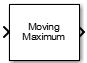Documentation

# Moving Maximum

Moving maximum

• Library:
• DSP System Toolbox / Statistics## Description

The Moving Maximum block determines the moving maximum of the input signal along each channel independently over time. The block uses the sliding window method to determine the moving maximum. In this method, a window of specified length moves over each channel sample by sample, and the block determines the maximum over the data in the window. For more details, see Algorithms.

## Ports

### Input

expand all

Data over which the moving maximum is determined using the sliding window method. The block accepts real-valued or complex-valued multichannel inputs, that is, m-by-n size inputs, where m ≥ 1 and n ≥ 1. The block also accepts variable-size inputs. During simulation, you can change the size of each input channel. However, the number of channels cannot change.

Data Types: `single` | `double`
Complex Number Support: Yes

### Output

expand all

Moving maximum output, determined using the sliding window method. The size of the output matches the size of the input. The window slides column-wise along each channel, and the block determines the maximum of the data in the window. For more details, see Algorithms.

Data Types: `single` | `double`
Complex Number Support: Yes

## Parameters

expand all

When you select this check box, the length of the sliding window is equal to the value you specify through the parameter. When you clear this check box, the length of the sliding window is infinite. In this mode, the block determines the maximum of the current sample and all previous samples in the channel.

Window length specifies the length of the sliding window. This parameter appears when you select the check box.

• `Code generation`

Simulate model using generated C code. The first time you run a simulation, Simulink® generates C code for the block. The C code is reused for subsequent simulations, as long as the model does not change. This option requires additional startup time but provides faster simulation speed than ```Interpreted execution```.

• `Interpreted execution`

Simulate model using the MATLAB®  interpreter. This option shortens startup time but has slower simulation speed than ```Code generation```.

## Block Characteristics

 Data Types `double` | `single` | `base integer` Multidimensional Signals `No` Variable-Size Signals `Yes`

expand all

Watch now